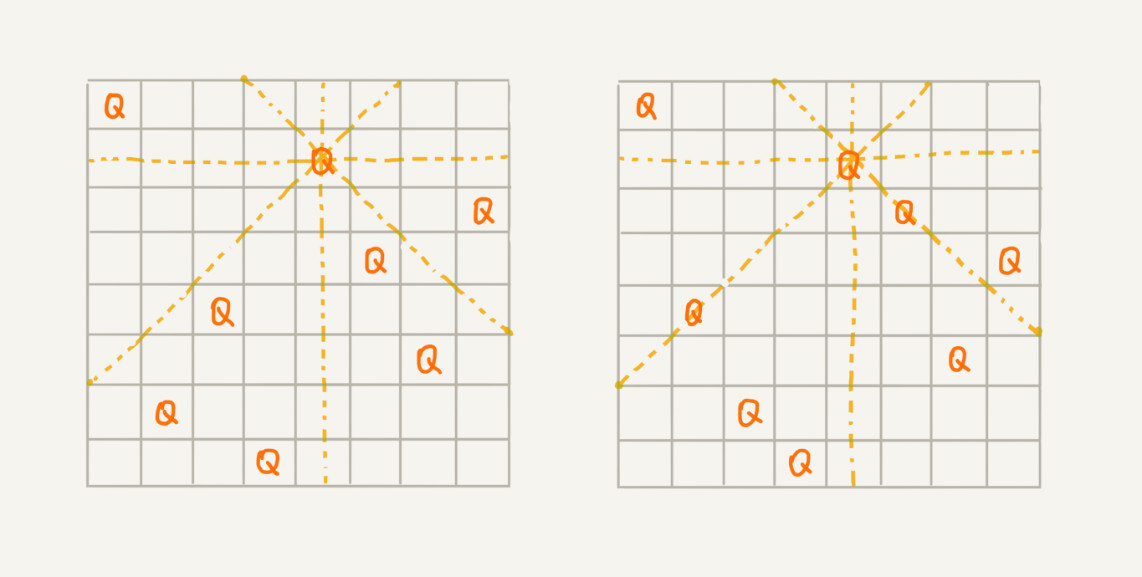## 如何理解“回溯算法”？

2004 年上映了一部非常著名的电影《蝴蝶效应》，讲的就是主人公为了达到自己的目标，一直通过回溯的方法，回到童年，在关键的岔路口，重新做选择。当然，这只是科幻电影，我们的人生是无法倒退的，但是这其中蕴含的思想其实就是回溯算法。```int[] result = new int;//全局或成员变量,下标表示行,值表示queen存储在哪一列
public void cal8queens(int row) { // 调用方式：cal8queens(0);
if (row == 8) { // 8个棋子都放置好了，打印结果
printQueens(result);
return; // 8行棋子都放好了，已经没法再往下递归了，所以就return
}
for (int column = 0; column < 8; ++column) { // 每一行都有8中放法
if (isOk(row, column)) { // 有些放法不满足要求
result[row] = column; // 第row行的棋子放到了column列
cal8queens(row+1); // 考察下一行
}
}
}

private boolean isOk(int row, int column) {//判断row行column列放置是否合适
int leftup = column - 1, rightup = column + 1;
for (int i = row-1; i >= 0; --i) { // 逐行往上考察每一行
if (result[i] == column) return false; // 第i行的column列有棋子吗？
if (leftup >= 0) { // 考察左上对角线：第i行leftup列有棋子吗？
if (result[i] == leftup) return false;
}
if (rightup < 8) { // 考察右上对角线：第i行rightup列有棋子吗？
if (result[i] == rightup) return false;
}
--leftup; ++rightup;
}
return true;
}

private void printQueens(int[] result) { // 打印出一个二维矩阵
for (int row = 0; row < 8; ++row) {
for (int column = 0; column < 8; ++column) {
if (result[row] == column) System.out.print("Q ");
else System.out.print("* ");
}
System.out.println();
}
System.out.println();
}```

## 两个回溯算法的经典应用

### 1.0-1 背包

0-1 背包是非常经典的算法问题，很多场景都可以抽象成这个问题模型。这个问题的经典解法是动态规划，不过还有一种简单但没有那么高效的解法，那就是今天讲的回溯算法。动态规划的解法我下一节再讲，我们先来看下，如何用回溯法解决这个问题。

0-1 背包问题有很多变体，我这里介绍一种比较基础的。我们有一个背包，背包总的承载重量是 Wkg。现在我们有 n 个物品，每个物品的重量不等，并且不可分割。我们现在期望选择几件物品，装载到背包中。在不超过背包所能装载重量的前提下，如何让背包中物品的总重量最大？

```public int maxW = Integer.MIN_VALUE; //存储背包中物品总重量的最大值
// cw表示当前已经装进去的物品的重量和；i表示考察到哪个物品了；
// w背包重量；items表示每个物品的重量；n表示物品个数
// 假设背包可承受重量100，物品个数10，物品重量存储在数组a中，那可以这样调用函数：
// f(0, 0, a, 10, 100)
public void f(int i, int cw, int[] items, int n, int w) {
if (cw == w || i == n) { // cw==w表示装满了;i==n表示已经考察完所有的物品
if (cw > maxW) maxW = cw;
return;
}
f(i+1, cw, items, n, w);
if (cw + items[i] <= w) {// 已经超过可以背包承受的重量的时候，就不要再装了
f(i+1,cw + items[i], items, n, w);
}
}```

### 2. 正则表达式

```public class Pattern {
private boolean matched = false;
private char[] pattern; // 正则表达式
private int plen; // 正则表达式长度

public Pattern(char[] pattern, int plen) {
this.pattern = pattern;
this.plen = plen;
}

public boolean match(char[] text, int tlen) { // 文本串及长度
matched = false;
rmatch(0, 0, text, tlen);
return matched;
}

private void rmatch(int ti, int pj, char[] text, int tlen) {
if (matched) return; // 如果已经匹配了，就不要继续递归了
if (pj == plen) { // 正则表达式到结尾了
if (ti == tlen) matched = true; // 文本串也到结尾了
return;
}
if (pattern[pj] == '*') { // *匹配任意个字符
for (int k = 0; k <= tlen-ti; ++k) {
rmatch(ti+k, pj+1, text, tlen);
}
} else if (pattern[pj] == '?') { // ?匹配0个或者1个字符
rmatch(ti, pj+1, text, tlen);
rmatch(ti+1, pj+1, text, tlen);
} else if (ti < tlen && pattern[pj] == text[ti]) { // 纯字符匹配才行
rmatch(ti+1, pj+1, text, tlen);
}
}
}```